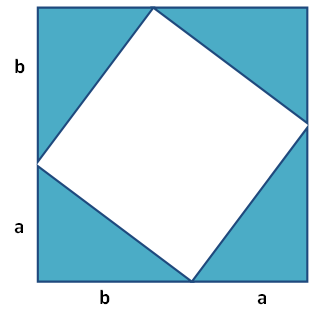A Pigeonhole Proof

Given $$n$$ objects and $$k < n$$ containers which hold them. Prove that at least one container has more than one object.

• Proof: Suppose not.
• (That means that $$k < n$$ and all containers have one or less objects.)
• Let $$n_i$$ be the number of objects in container $$i$$.
• Then $$n = n_1 + \ldots + n_k$$.
• For each $$i$$ we know $$n_i \leq 1$$ (based on our supposition)
• Thus $$n = n_1 + \ldots + n_k \leq k < n$$.
• That implies that $$n < n$$ which is a contradiction.

If $$x^3$$ is irrational then $$x$$ is irrational.

But $$m^3, n^3 \in \mathbb{Z}$$ and $$n^3 \neq 0$$ so $$x^3$$ is rational.

Let $$n$$ be an integer. Prove that $$9n^2 + 3n -2$$ is even.

• Proof: Solve first for evens, then for odds.
• EVENS: Let $$n = 2k$$ for some $$k$$.
• $$9 (2k)^2 + 3(2k) -2 = 36k^2 + 6k - 2$$
• $$= 2(18k^2 + 3k -1)$$ which is even.
• ODDS: Let $$n = 2k + 1$$ for some $$k$$.
• We explore: $$9 (2k + 1)^2 + 3(2k + 1) -2$$
• $$= 36k^2 + 36k + 9 + 6k + 3 - 2$$
• $$= 36k^2 + 42k + 10 = 2(18k^2 + 21k + 5)$$ even

ICUP

Show that $$\sqrt{2}$$ is irrational

• Proof: Suppose not.
• Let $$\sqrt{2} = \frac{m}{n}$$
• Without loss of generality we can say only one of $$m$$ and $$n$$ are even (remove common factors)
• Now $$2 = \frac{m^2}{n^2}$$ which is $$2n^2 = m^2$$
• That means that $$m^2$$ is even.
• An odd number squared is odd, so $$m = 2k$$ for some $$k$$.
• Replacing: $$2n^2 = 4k^2$$.
• Simplifying: $$n^2 = 2k^2$$.
• This implies that $$n$$ is also even.

Pythagorean Theorem $$a^2 + b^2 = c^2$$